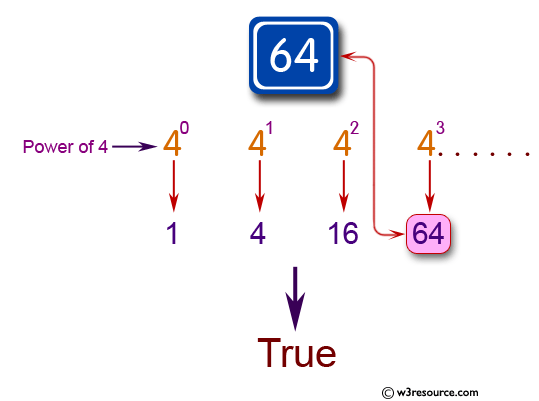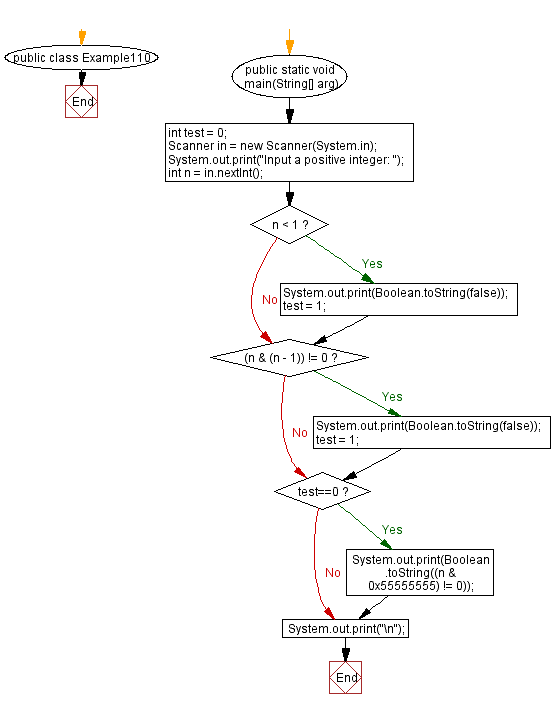﻿ Java: Check whether a given integer is a power of 4 or not# Java Exercises: Check whether a given integer is a power of 4 or not

## Java Basic: Exercise-110 with Solution

Write a Java program to check whether an given integer is a power of 4 or not.

Given num = 64, return true. Given num = 6, return false.

Pictorial Presentation:Sample Solution:

Java Code:

``````import java.util.Scanner;
public class Example110 {
public static void main(String[] arg)
{
int test = 0;
Scanner in = new Scanner(System.in);
System.out.print("Input a positive integer: ");
int n = in.nextInt();
if (n < 1) {
System.out.print(Boolean.toString(false));
test = 1;
}
if ((n & (n - 1)) != 0) {
System.out.print(Boolean.toString(false));
test = 1;
}
if (test==0)
{
System.out.print(Boolean.toString((n & 0x55555555) != 0));
}
System.out.print("\n");
}
}
```
```

Sample Output:

```Input a positive integer: 64
true
```

Flowchart:Java Code Editor:

What is the difficulty level of this exercise?

Test your Programming skills with w3resource's quiz.

﻿

## Java: Tips of the Day

getEnumMap

Converts to enum to Map where key is the name and value is Enum itself.

```public static <E extends Enum<E>> Map<String, E> getEnumMap(final Class<E> enumClass) {
return Arrays.stream(enumClass.getEnumConstants())
.collect(Collectors.toMap(Enum::name, Function.identity()));
}
```

Ref: https://bit.ly/3xXcFZt